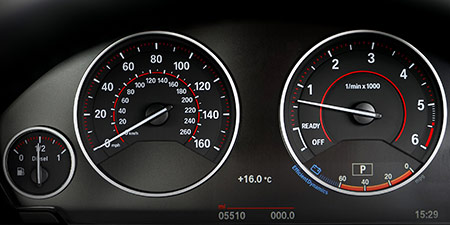# Speed of sound to Miles per hour (mph)

Units of speed ﹣ Converter Speed of sound to Miles per hour

Here you can convert the Speed unit Speed of sound into the unit Miles per hour and vice versa you can convert Miles per hour into Speed of sound. By clicking the "Swap units" icon, you will always obtain the desired conversion in the calculation result. With the following calculator you can also calculate any other Speed unit.

## Info about "Speed of sound"

Definition: If an object travels at the speed of sound in air at 20 degrees Celsius for one second, it travels a distance of 343 meters per second. Actually, it is equivalent to 1.234,8 km/h.

The speed of sound is often illustrated by an example of a thunderstorm: if lightning strikes within 3 seconds of the thunder sound, then the thunderstorm is about 1 kilometer away. Another example, in a 100-meter sprint, the starting shot is fired directly next to the starting blocks. Imagine, if the start shot was fired at the finish line, it would take about 0.3 seconds of time to hear the shot in the start blocks.

## Info about "Miles per hour"

The unit of length known as a "mile" and it should not be confused with the nautical mile and very commonly used in the Anglo-American countries. Therefore, the metric system is defined in miles (instead of kilometers) per time unit. For example, if a vehicle travels at a speed of 100 miles per hour (100 mph), it will move around 160 km per hour in the metric system.

The units are defined as follows: An object moving at 1 "mph" per hour will cover a distance of one mile. Therefore, 1 mile is equivalent to 1.609344 kilometers.

## Formula for the conversion of Speed of sound to Miles per hour (mph) and vice versa

The calculation from Speed of sound to Miles per hour shall be made using the following conversion formula:

Conversion formula Speed of sound to Miles per hour

Determine the number of Miles per hour from Speed of sound

Speed of sound × 767.26914848336

## Formula for the conversion of Miles per hour (mph) to Speed of sound

The calculation from Miles per hour to Speed of sound shall be made using the following conversion formula:

Conversion formula Miles per hour to Speed of sound

Determine the number of Speed of sound from Miles per hour

Miles per hour × 0.001303323614636

## Overview table : How many Speed of sound are in a Miles per hour ?

Speed of sound ⇒ Miles per hour mph
0.01   are  7.67269 mph
0.02   are  15.34538 mph
0.03   are  23.01807 mph
0.04   are  30.69076 mph
0.05   are  38.36345 mph
0.06   are  46.03614 mph
0.07   are  53.70884 mph
0.08   are  61.38153 mph
0.09   are  69.05422 mph
0.10   are  76.72691 mph
0.20   are  153.45383 mph
0.30   are  230.18074 mph
0.40   are  306.90765 mph
0.50   are  383.63457 mph
0.60   are  460.36148 mph
0.70   are  537.08840 mph
0.80   are  613.81531 mph
0.90   are  690.54223 mph
1   corresponds to  767.26914 mph
2   are  1 534.53829 mph
3   are  2 301.80744 mph
4   are  3 069.07659 mph
5   are  3 836.34574 mph
6   are  4 603.61489 mph
7   are  5 370.88403 mph
8   are  6 138.15318 mph
9   are  6 905.42233 mph
10   are  7 672.69148 mph
20   are  15 345.38297 mph
30   are  23 018.07445 mph
40   are  30 690.76593 mph
50   are  38 363.45742 mph
60   are  46 036.14890 mph
70   are  53 708.84039 mph
80   are  61 381.53187 mph
90   are  69 054.22336 mph
100   are  76 726.91484 mph
200   are  153 453.82969 mph
300   are  230 180.74454 mph
400   are  306 907.65939 mph
500   are  383 634.57424 mph
600   are  460 361.48909 mph
700   are  537 088.40393 mph
800   are  613 815.31878 mph
900   are  690 542.23363 mph
1 000   are  767 269.14848 mph

## Overview table : How many Miles per hour are in a Speed of sound ?

Miles per hour mph ⇒ Speed of sound
0.01 mph  are  0.00001
0.02 mph  are  0.00002
0.03 mph  are  0.00003
0.04 mph  are  0.00005
0.05 mph  are  0.00006
0.06 mph  are  0.00007
0.07 mph  are  0.00009
0.08 mph  are  0.00010
0.09 mph  are  0.00011
0.10 mph  are  0.00013
0.20 mph  are  0.00026
0.30 mph  are  0.00039
0.40 mph  are  0.00052
0.50 mph  are  0.00065
0.60 mph  are  0.00078
0.70 mph  are  0.00091
0.80 mph  are  0.00104
0.90 mph  are  0.00117
1 mph  corresponds to  0.00130
2 mph  are  0.00260
3 mph  are  0.00391
4 mph  are  0.00521
5 mph  are  0.00651
6 mph  are  0.00782
7 mph  are  0.00912
8 mph  are  0.01042
9 mph  are  0.01173
10 mph  are  0.01303
20 mph  are  0.02606
30 mph  are  0.03910
40 mph  are  0.05213
50 mph  are  0.06516
60 mph  are  0.07819
70 mph  are  0.09123
80 mph  are  0.10426
90 mph  are  0.11729
100 mph  are  0.13033
200 mph  are  0.26066
300 mph  are  0.39099
400 mph  are  0.52132
500 mph  are  0.65166
600 mph  are  0.78199
700 mph  are  0.91232
800 mph  are  1.04265
900 mph  are  1.17299
1 000 mph  are  1.30332

## Source information

As source for the information in the 'Units of speed' category, we have used in particular:

## Last update on February 19, 2023

The pages of the 'Units of speed' category were last editorially reviewed by Stefan Banse on February 19, 2023. They all correspond to the current status.

### Previous changes on October 9, 2021

• November 9, 2020: Publication of the Speed converter
• Editorial revision of all texts in this category